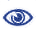# The Prague Stringology Conference 2009

An input sensitive online algorithm for LCS computation

 Abstract: We consider the classic problem of computing (the length of) the longest common subsequence (LCS) between two strings A and B with lengths m and n, respectively. There are several input sensitive algorithms for this problem, such as the O(σn + min{Lm,L(n-L)}) algorithms by Rick (1995) and Goeman and Clausen (2002) and the O(σn + min{σd,Lm}) algorithms by Chin and Poon (1990) and Rick (1995). Here L is the length of the LCS and d is the number of dominant matches between A and B, and σ is the alphabet size. These algorithms require O(σn) time preprocessing for both A and B. We propose a new fairly simple O(σm + min{Lm,L(n-L)}) time algorithm that works in online manner: It needs to preprocess only A, and it can process B one character at a time, without knowing the whole string B beforehand. The algorithm also adapts well to the linear space scheme of Hirschberg (1975) for recovering the LCS, which was not as easy with the above-mentioned algorithms. In addition, our scheme fits well into the context of incremental string comparison (LMZ 2007, IIST 2005). The original algorithm of Landau et al. (LMZ 2007) for this problem uses O(σm + Lm) space. By using our scheme instead, the space usage becomes O(σm + min{Lm,L(n-L)}).

 Download paper:PostScript PDF BibTeX reference
 Download presentation: